# RD Sharma Solutions for Class 11 Maths Chapter 21 Some Special Series

## RD Sharma Solutions Class 11 Maths Chapter 21 – Get Free PDF Updated for 2021-22

RD Sharma Solutions for Class 11 Maths Chapter 21 – Some Special Series are provided here for students to score good marks in the board exams. In this chapter, we intend to discuss the sum to ‘n’ terms of some other special series viz. series of natural numbers, series of square of natural numbers, series of cubes of natural numbers, etc. RD Sharma Solutions are basically designed for CBSE students, in accordance with the latest syllabus prescribed by the CBSE Board.

Our expert team has designed these solutions in an easily understandable language. The practice is an essential task to learn and score well in Mathematics. The pdfs of RD Sharma Class 11 Maths Solutions are provided here. Students are required to go through RD Sharma Solutions thoroughly before the final exams to score well and intensify their problem-solving abilities.

Chapter 21 – Some Special Series contains two exercises and the RD Sharma Solutions present in this page provide solutions to the questions present in each exercise. Now, let us have a look at the concepts discussed in this chapter.

• Sum to ‘n’ terms of some special series.
• Sum of the first ‘n’ natural numbers.
• Sum of the squares of first ‘n’ natural numbers.
• Sum of the cubes of first ‘n’ natural numbers.
• Method of difference.
• Summation of some special series.

## Download the pdf of RD Sharma Solutions for Class 11 Maths Chapter 21 – Some Special Series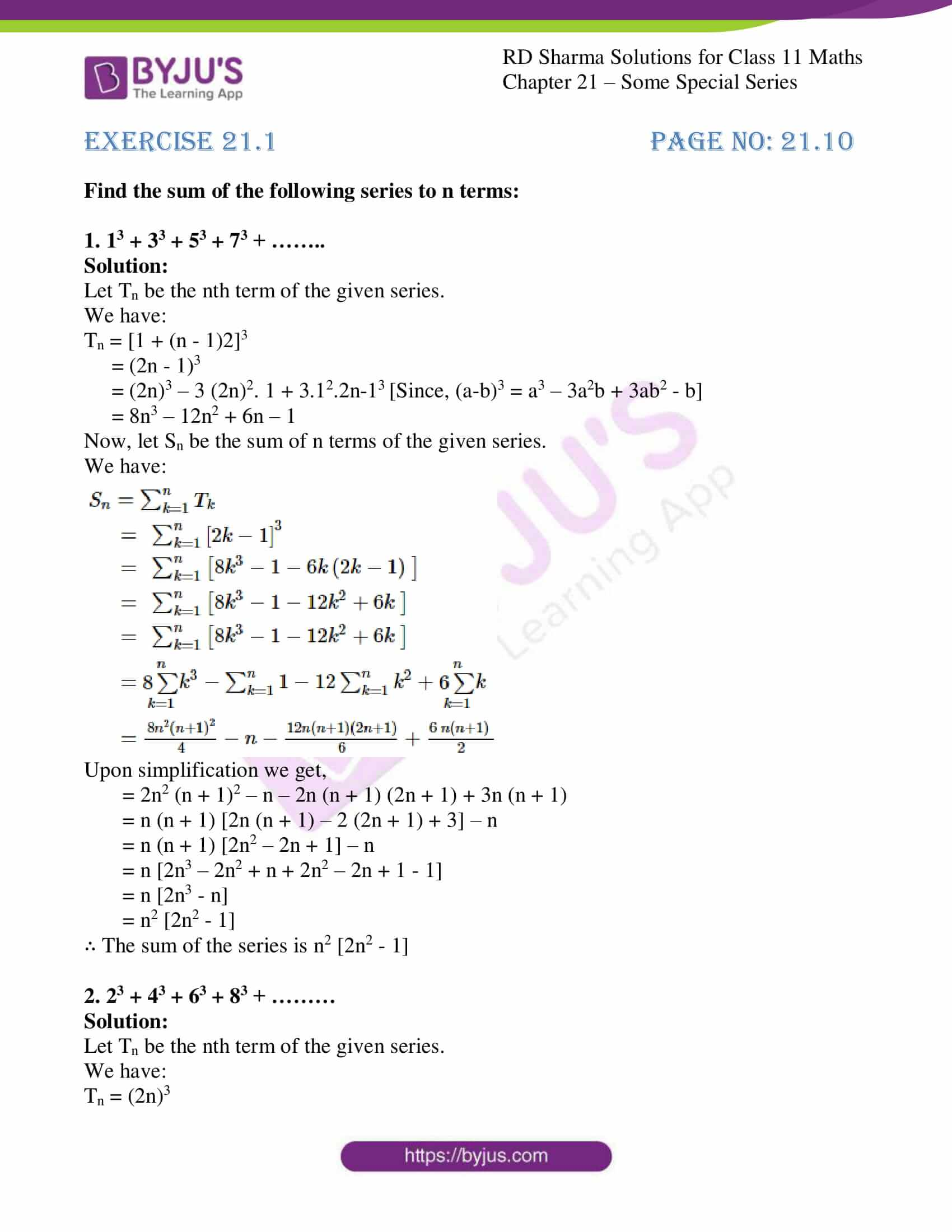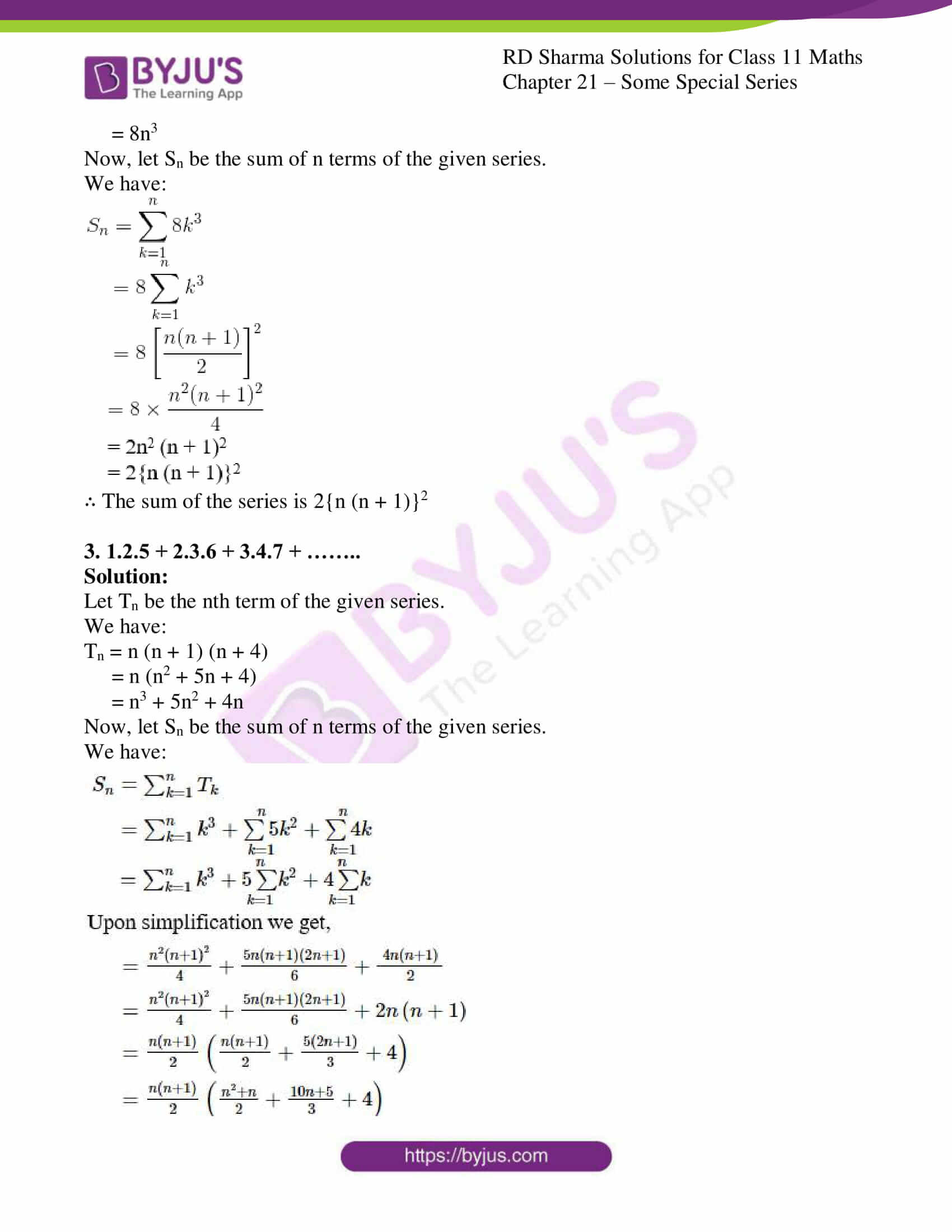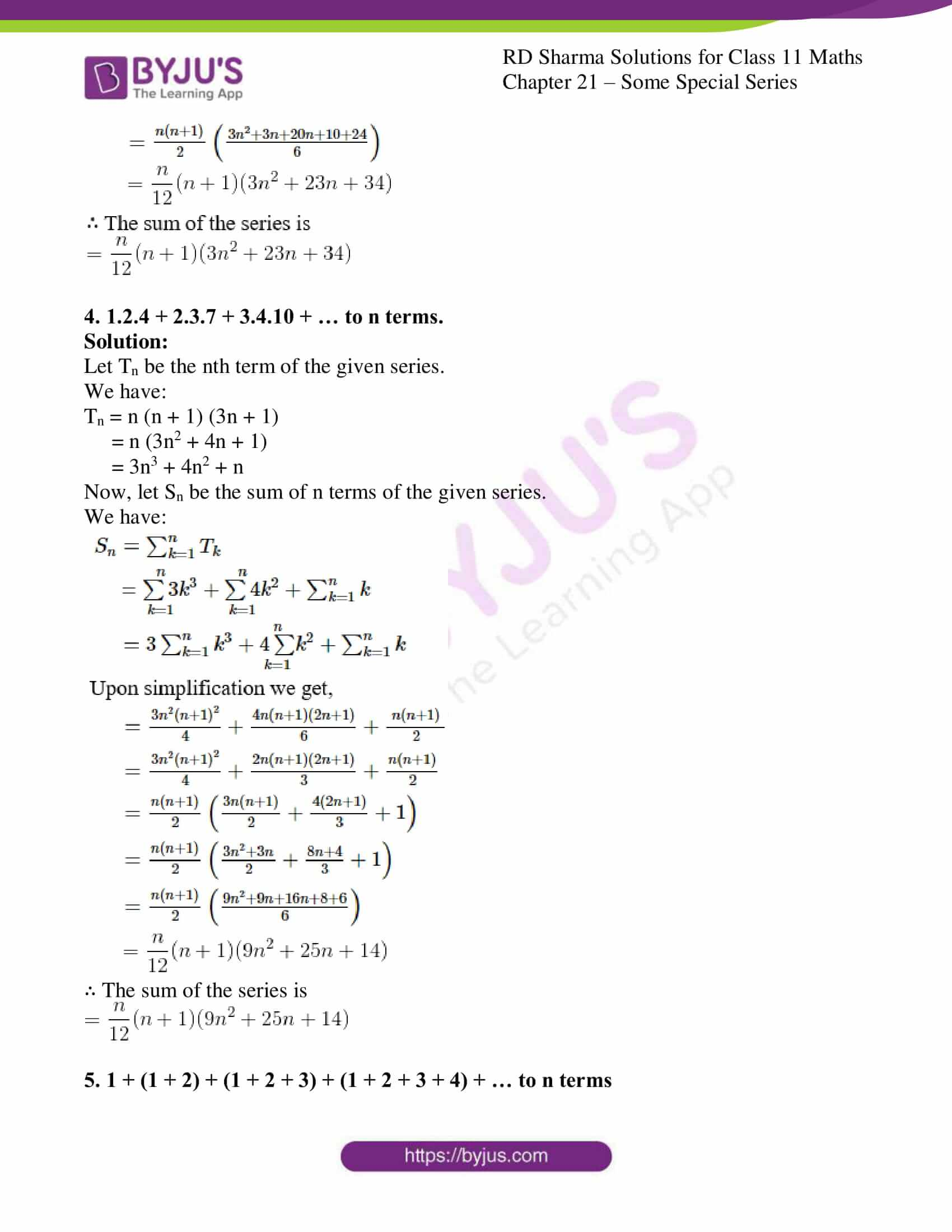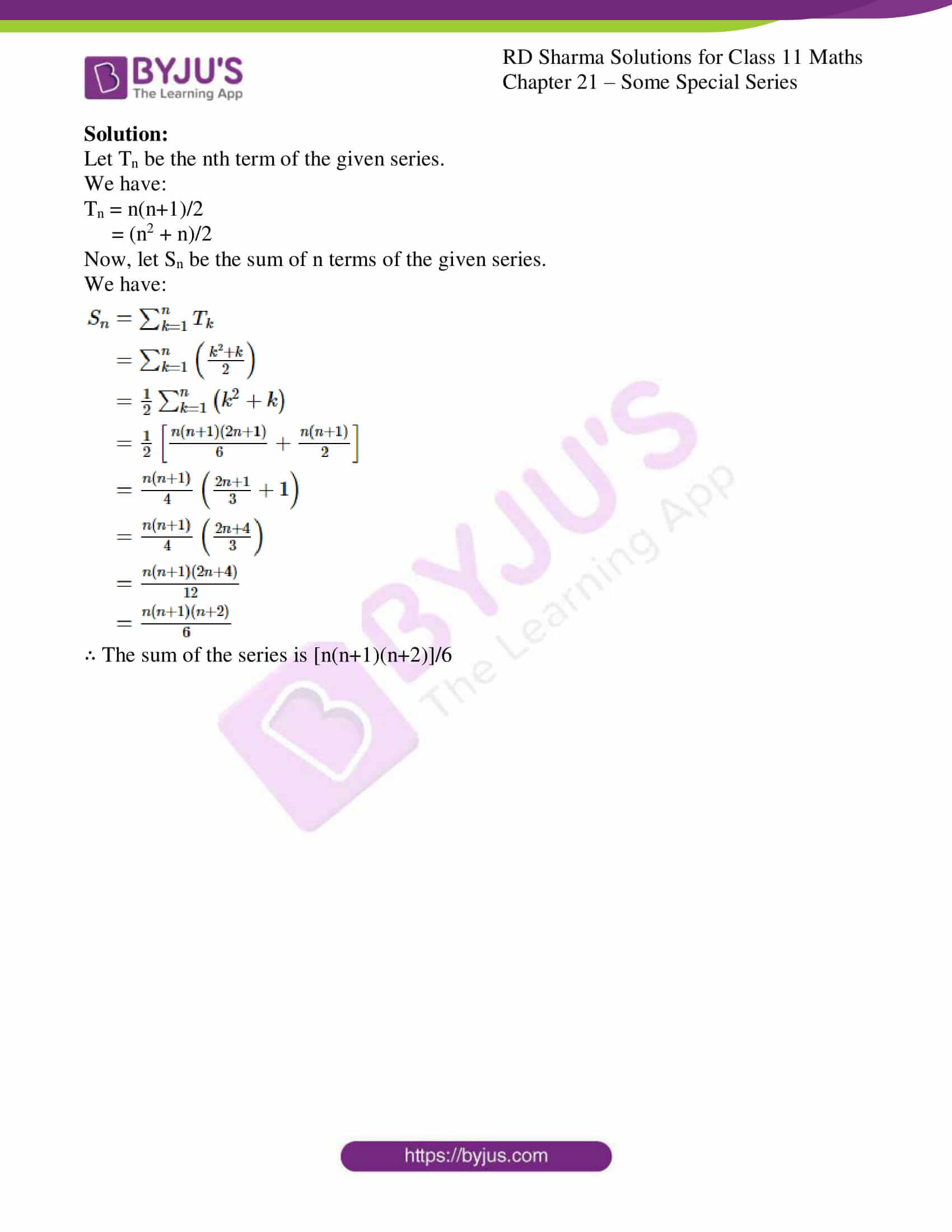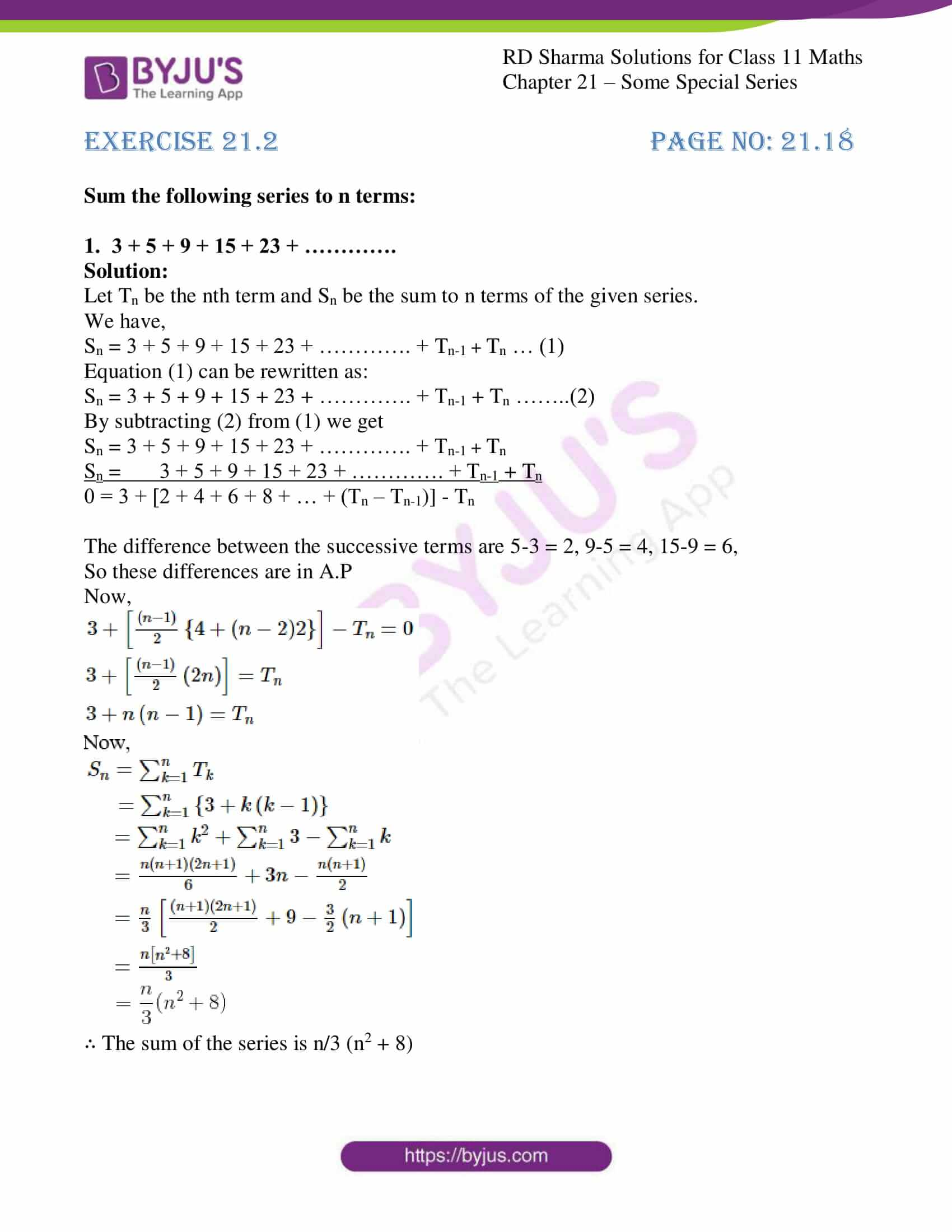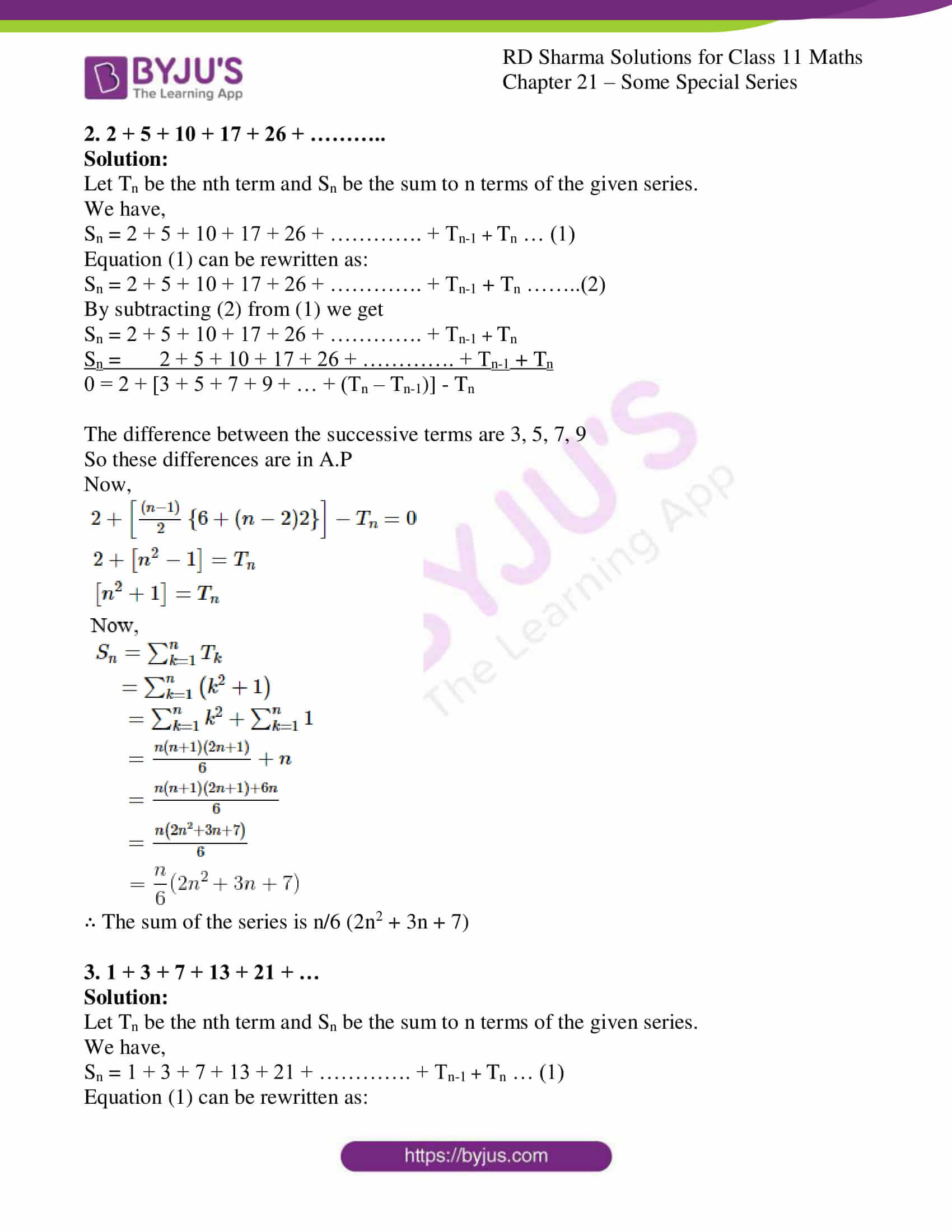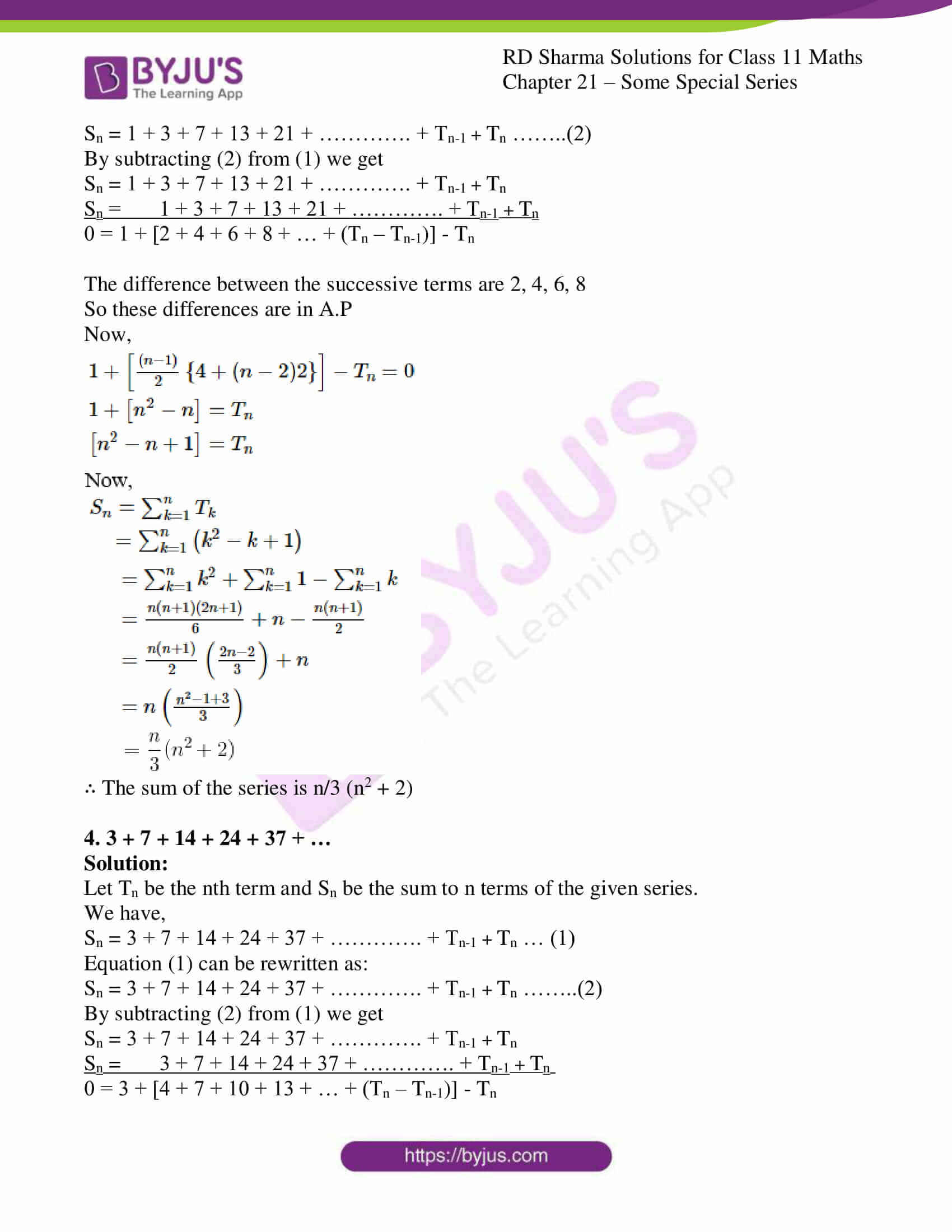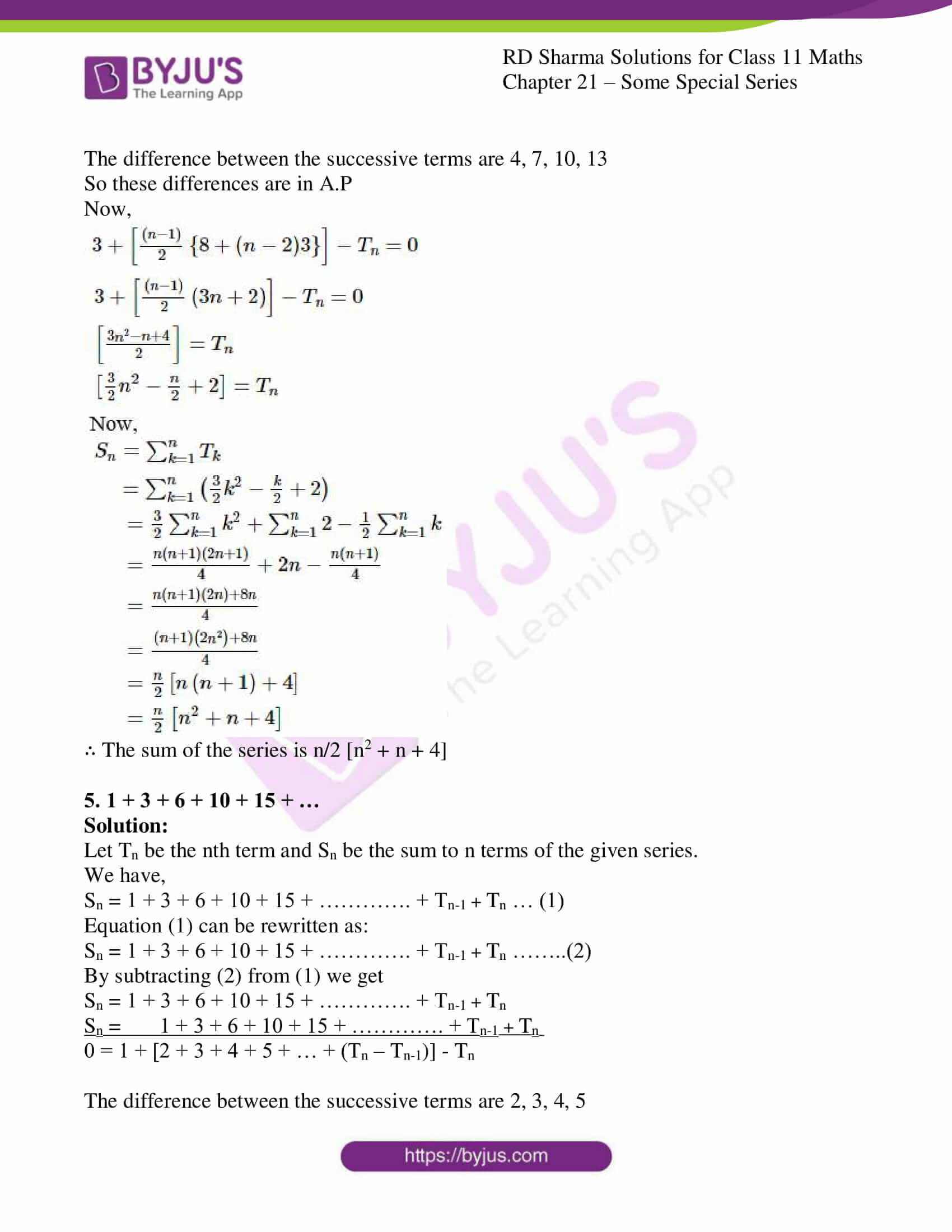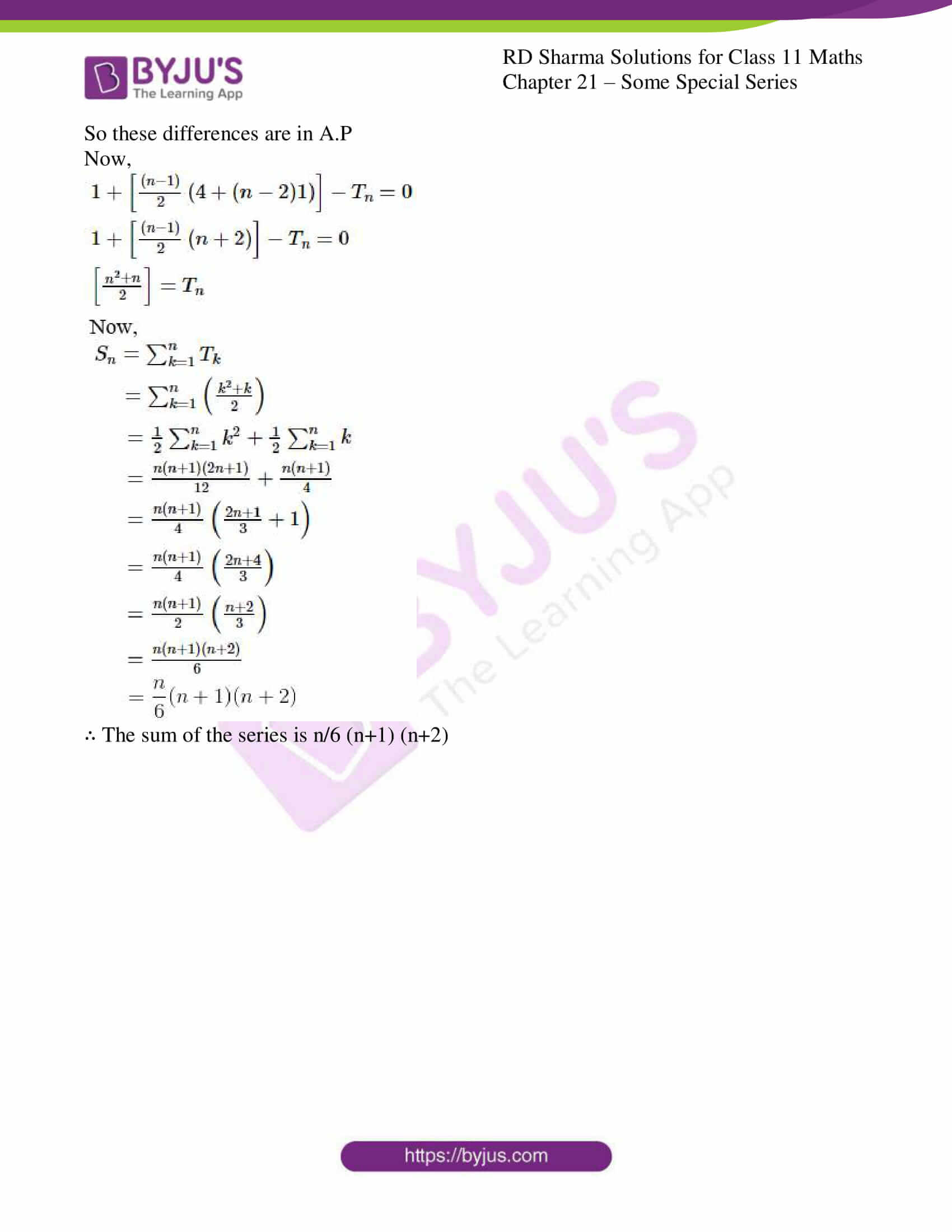### Access answers to RD Sharma Solutions for Class 11 Maths Chapter 21 – Some Special Series

EXERCISE 21.1 PAGE NO: 21.10

Find the sum of the following series to n terms:

1. 13 + 33 + 53 + 73 + ……..

Solution:

Let Tn be the nth term of the given series.

We have:

Tn = [1 + (n – 1)2]3

= (2n – 1)3

= (2n)3 – 3 (2n)2. 1 + 3.12.2n-13 [Since, (a-b)3 = a3 – 3a2b + 3ab2 – b]

= 8n3 – 12n2 + 6n – 1

Now, let Sn be the sum of n terms of the given series.

We have: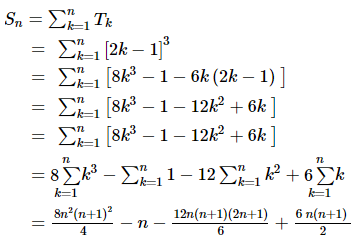Upon simplification we get,

= 2n2 (n + 1)2 – n – 2n (n + 1) (2n + 1) + 3n (n + 1)

= n (n + 1) [2n (n + 1) – 2 (2n + 1) + 3] – n

= n (n + 1) [2n2 – 2n + 1] – n

= n [2n3 – 2n2 + n + 2n2 – 2n + 1 – 1]

= n [2n3 – n]

= n2 [2n2 – 1]

∴ The sum of the series is n2 [2n2 – 1]

2. 23 + 43 + 63 + 83 + ………

Solution:

Let Tn be the nth term of the given series.

We have:

Tn = (2n)3

= 8n3

Now, let Sn be the sum of n terms of the given series.

We have: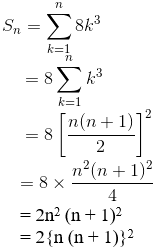∴ The sum of the series is 2{n (n + 1)}2

3. 1.2.5 + 2.3.6 + 3.4.7 + ……..

Solution:

Let Tn be the nth term of the given series.

We have:

Tn = n (n + 1) (n + 4)

= n (n2 + 5n + 4)

= n3 + 5n2 + 4n

Now, let Sn be the sum of n terms of the given series.

We have: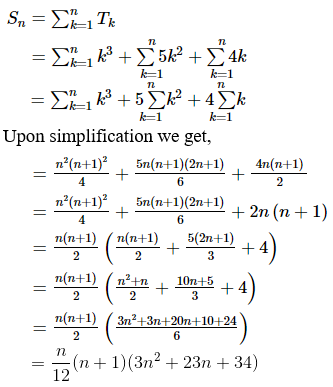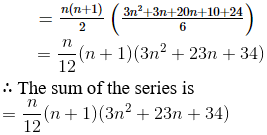4. 1.2.4 + 2.3.7 + 3.4.10 + … to n terms.

Solution:

Let Tn be the nth term of the given series.

We have:

Tn = n (n + 1) (3n + 1)

= n (3n2 + 4n + 1)

= 3n3 + 4n2 + n

Now, let Sn be the sum of n terms of the given series.

We have: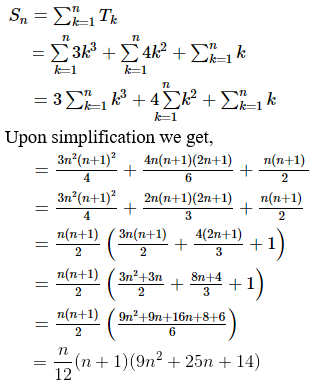∴ The sum of the series is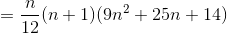5. 1 + (1 + 2) + (1 + 2 + 3) + (1 + 2 + 3 + 4) + … to n terms

Solution:

Let Tn be the nth term of the given series.

We have:

Tn = n(n+1)/2

= (n2 + n)/2

Now, let Sn be the sum of n terms of the given series.

We have: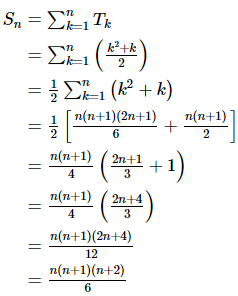∴ The sum of the series is [n(n+1)(n+2)]/6

EXERCISE 21.2 PAGE NO: 21.18

Sum the following series to n terms:

1. 3 + 5 + 9 + 15 + 23 + ………….

Solution:

Let Tn be the nth term and Sn be the sum to n terms of the given series.

We have,

Sn = 3 + 5 + 9 + 15 + 23 + …………. + Tn-1 + Tn … (1)

Equation (1) can be rewritten as:

Sn = 3 + 5 + 9 + 15 + 23 + …………. + Tn-1 + Tn ……..(2)

By subtracting (2) from (1) we get

Sn = 3 + 5 + 9 + 15 + 23 + …………. + Tn-1 + Tn

Sn = 3 + 5 + 9 + 15 + 23 + …………. + Tn-1 + Tn

0 = 3 + [2 + 4 + 6 + 8 + … + (Tn – Tn-1)] – Tn

The difference between the successive terms are 5-3 = 2, 9-5 = 4, 15-9 = 6,

So these differences are in A.P

Now,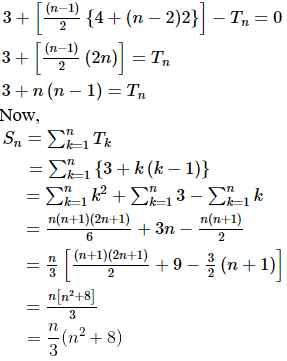∴ The sum of the series is n/3 (n2 + 8)

2. 2 + 5 + 10 + 17 + 26 + ………..

Solution:

Let Tn be the nth term and Sn be the sum to n terms of the given series.

We have,

Sn = 2 + 5 + 10 + 17 + 26 + …………. + Tn-1 + Tn … (1)

Equation (1) can be rewritten as:

Sn = 2 + 5 + 10 + 17 + 26 + …………. + Tn-1 + Tn ……..(2)

By subtracting (2) from (1) we get

Sn = 2 + 5 + 10 + 17 + 26 + …………. + Tn-1 + Tn

Sn = 2 + 5 + 10 + 17 + 26 + …………. + Tn-1 + Tn

0 = 2 + [3 + 5 + 7 + 9 + … + (Tn – Tn-1)] – Tn

The difference between the successive terms are 3, 5, 7, 9

So these differences are in A.P

Now,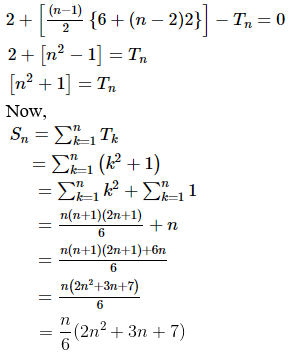∴ The sum of the series is n/6 (2n2 + 3n + 7)

3. 1 + 3 + 7 + 13 + 21 + …

Solution:

Let Tn be the nth term and Sn be the sum to n terms of the given series.

We have,

Sn = 1 + 3 + 7 + 13 + 21 + …………. + Tn-1 + Tn … (1)

Equation (1) can be rewritten as:

Sn = 1 + 3 + 7 + 13 + 21 + …………. + Tn-1 + Tn ……..(2)

By subtracting (2) from (1) we get

Sn = 1 + 3 + 7 + 13 + 21 + …………. + Tn-1 + Tn

Sn = 1 + 3 + 7 + 13 + 21 + …………. + Tn-1 + Tn

0 = 1 + [2 + 4 + 6 + 8 + … + (Tn – Tn-1)] – Tn

The difference between the successive terms are 2, 4, 6, 8

So these differences are in A.P

Now,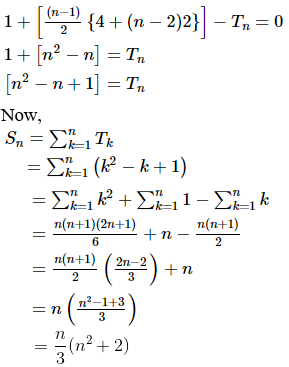∴ The sum of the series is n/3 (n2 + 2)

4. 3 + 7 + 14 + 24 + 37 + …

Solution:

Let Tn be the nth term and Sn be the sum to n terms of the given series.

We have,

Sn = 3 + 7 + 14 + 24 + 37 + …………. + Tn-1 + Tn … (1)

Equation (1) can be rewritten as:

Sn = 3 + 7 + 14 + 24 + 37 + …………. + Tn-1 + Tn ……..(2)

By subtracting (2) from (1) we get

Sn = 3 + 7 + 14 + 24 + 37 + …………. + Tn-1 + Tn

Sn = 3 + 7 + 14 + 24 + 37 + …………. + Tn-1 + Tn

0 = 3 + [4 + 7 + 10 + 13 + … + (Tn – Tn-1)] – Tn

The difference between the successive terms are 4, 7, 10, 13

So these differences are in A.P

Now,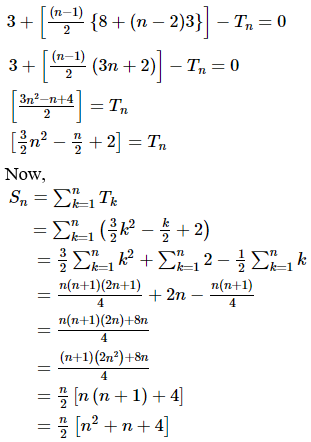∴ The sum of the series is n/2 [n2 + n + 4]

5. 1 + 3 + 6 + 10 + 15 + …

Solution:

Let Tn be the nth term and Sn be the sum to n terms of the given series.

We have,

Sn = 1 + 3 + 6 + 10 + 15 + …………. + Tn-1 + Tn … (1)

Equation (1) can be rewritten as:

Sn = 1 + 3 + 6 + 10 + 15 + …………. + Tn-1 + Tn ……..(2)

By subtracting (2) from (1) we get

Sn = 1 + 3 + 6 + 10 + 15 + …………. + Tn-1 + Tn

Sn = 1 + 3 + 6 + 10 + 15 + …………. + Tn-1 + Tn

0 = 1 + [2 + 3 + 4 + 5 + … + (Tn – Tn-1)] – Tn

The difference between the successive terms are 2, 3, 4, 5

So these differences are in A.P

Now,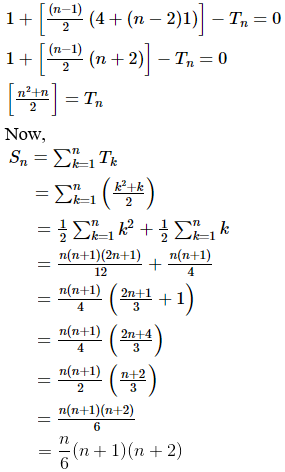∴ The sum of the series is n/6 (n+1) (n+2)

### Also, access exercises of RD Sharma Solutions for Class 11 Maths Chapter 21 – Some Special Series

Exercise 21.1 Solutions

Exercise 21.2 Solutions

## Frequently Asked Questions on RD Sharma Solutions for Class 11 Maths Chapter 21

### List the advantages of using the RD Sharma Solutions for Class 11 Maths Chapter 21?

The advantages of using the RD Sharma Solutions for Class 11 Maths Chapter 21 are:
1. Most reliable reference guide for exam preparation of students.
2. Fundamental concepts are well explained in a simple and understandable language.
3. Illustrations and graphs are included in the solutions to aid visual learning.
4. The solutions strictly adheres to the latest CBSE syllabus and guidelines.

### Can students depend on the RD Sharma Solutions for Class 11 Maths Chapter 21 for their exam preparations from BYJU’S ?

Yes, students can confidently depend on the RD Sharma Solutions for Class 11 Maths Chapter 21 by BYJU’S tutors since subject matter experts have prepared the solutions following the latest CBSE guidelines and exam pattern. Further, students can revise the important topics of the chapter quickly at any time and anywhere in both online and offline modes.

### What are the ways to learn the topics from RD Sharma Solutions for Class 11 Maths Chapter 21 faster?

Students can quickly comprehend the key topics by referring to the RD Sharma Solutions for Class 11 Maths Chapter 21. It comprises solutions to textbook questions solved in an elaborate manner highlighting the important concepts. Further, all the solutions are formulated by subject experts as per the latest CBSE syllabus and guidelines.